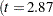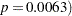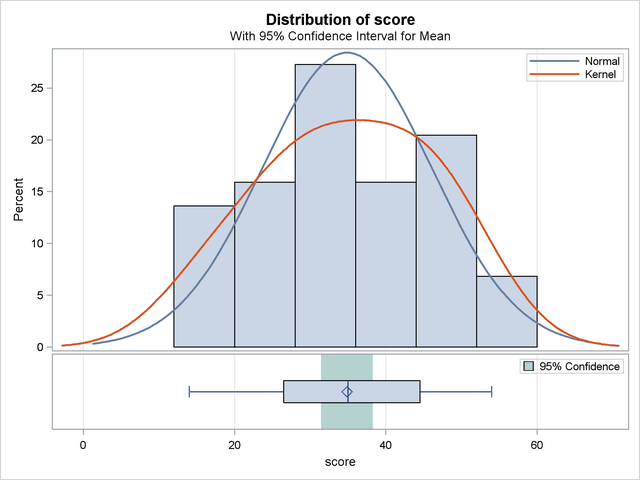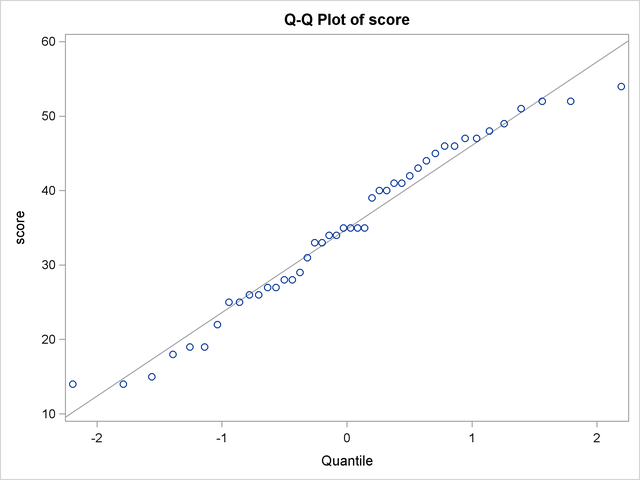## Example 95.2 One-Sample Comparison with the FREQ Statement

This example examines children’s reading skills. The data consist of Degree of Reading Power (DRP) test scores from 44 third-grade children and are taken from Moore (1995, p. 337). Their scores are given in the following DATA step:

```data read;
input score count @@;
datalines;
40 2   47 2   52 2   26 1   19 2
25 2   35 4   39 1   26 1   48 1
14 2   22 1   42 1   34 2   33 2
18 1   15 1   29 1   41 2   44 1
51 1   43 1   27 2   46 2   28 1
49 1   31 1   28 1   54 1   45 1
;
run;
```

The following statements invoke the TTEST procedure to test if the mean test score is equal to 30.

```ods graphics on;

var score;
freq count;
run;

ods graphics off;
```

The count variable contains the frequency of occurrence of each test score; this is specified in the FREQ statement. The output, shown in Output 95.2.1, contains the results.

Output 95.2.1 TTEST Results
The TTEST Procedure

Variable: score

N Mean Std Dev Std Err Minimum Maximum
44 34.8636 11.2303 1.6930 14.0000 54.0000

Mean 95% CL Mean Std Dev 95% CL Std Dev
34.8636 31.4493 38.2780 11.2303 9.2788 14.2291

DF t Value Pr > |t|
43 2.87 0.0063

The SAS log states that 30 observations and two variables have been read. However, the sample size given in the TTEST output is N=44. This is due to specifying the count variable in the FREQ statement. The test is significant,at the 5% level, so you can conclude that the mean test score is different from 30.

The summary panel in Output 95.2.2 shows a histogram with overlaid normal and kernel densities, a box plot, and the 95% confidence interval for the mean.

Output 95.2.2 Summary PanelThe Q-Q plot in Output 95.2.3 assesses the normality assumption.

Output 95.2.3 Q-Q PlotThe tight clustering of the points around the diagonal line is consistent with the normality assumption. You could use the UNIVARIATE procedure with the NORMAL option to numerically check the normality assumption.